# Models - Support Vector Machine

## Discuss support vector machine and more. One model every week.

Posted by Shubham Chaudhary on June 3, 2018

Classifying data is a common task in machine learning. Suppose some given data points each belong to one of two classes, and the goal is to decide which class a new data point will be in. In the case of support vector machines, a data point is viewed as a p-dimensional vector (a list of p numbers), and we want to know whether we can separate such points with a (p-1)-dimensional hyperplane. This is called a linear classifier.

There are many hyperplanes that might classify the data. One reasonable choice as the best hyperplane is the one that represents the largest separation, or margin, between the two classes. So we choose the hyperplane so that the distance from it to the nearest data point on each side is maximized.

If such a hyperplane exists, it is known as the maximum-margin hyperplane and the linear classifier it defines is known as a maximum margin classifier; or equivalently, the perceptron of optimal stability.

#### Usages

• Classification
• Regression
###### Pros
• Accuracy
• Works well on smaller cleaner datasets
• It can be more efficient because it uses a subset of training points
###### Cons
• Isn’t suited to larger datasets as the training time with SVMs can be high
• Less effective on noisier datasets with overlapping classes

### Maximum Margin

Refer to this lecture by MIT OCW

### Kernel Trick

In addition to performing linear classification, SVMs can efficiently perform a non-linear classification using what is called the kernel trick, implicitly mapping their inputs into high-dimensional feature spaces.

##### How to Select Support Vector Machine Kernels

When to use linear: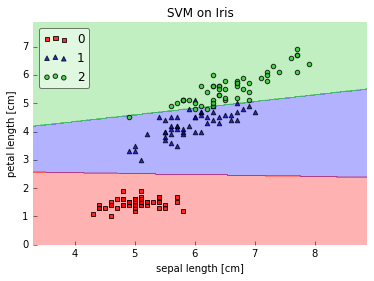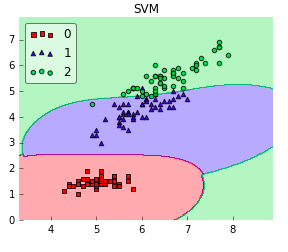When to use rbf: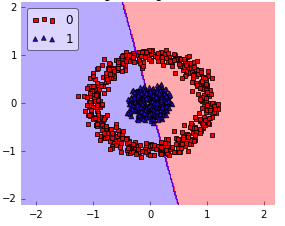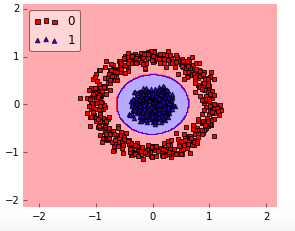How rbf did this?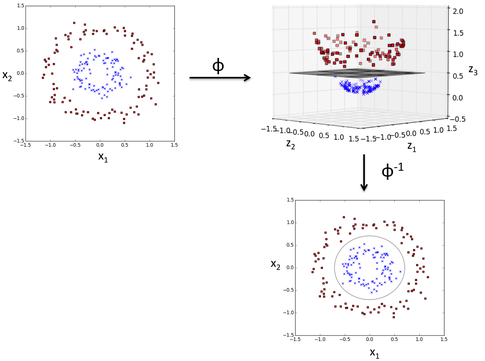The RBF kernel SVM decision region is actually also a linear decision region. What RBF kernel SVM actually does is to create non-linear combinations of your features to uplift your samples onto a higher-dimensional feature space where you can use a linear decision boundary to separate your classes: